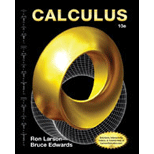# The probability that a randomly selected warbler has a length between a and b centimeters is given as, P ( a ≤ x ≤ b ) = 1 0.95 2 π ∫ a b e − ( x − 12.9 ) 2 1.805 d x . The probability that a randomly selected warbler has a length of 13 centimeters or greater.### Calculus

10th Edition
Ron Larson + 1 other
Publisher: Cengage Learning
ISBN: 9781285057095### Calculus

10th Edition
Ron Larson + 1 other
Publisher: Cengage Learning
ISBN: 9781285057095

#### Solutions

Chapter 8, Problem 91RE

(a)

To determine

## To calculate: The probability that a randomly selected warbler has a length between a and b centimeters is given as, P(a≤x≤b)=10.952π∫abe−(x−12.9)21.805dx. The probability that a randomly selected warbler has a length of 13 centimeters or greater.

(b)

To determine

### Want to see the full answer?

Check out a sample textbook solution.

### Want to see this answer and more?

Experts are waiting 24/7 to provide step-by-step solutions in as fast as 30 minutes!*

*Response times may vary by subject and question complexity. Median response time is 34 minutes for paid subscribers and may be longer for promotional offers.